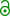Geometric mean and CV [Power / Sample Size]

Hi Rocco,

» […] is it even reasonable to use the geometric CV for the reference? Isn’t the CV you want to input into a sample calculation the CV corresponding to the difference of the Test and Reference? Since you do not have the Test group here, I am a bit confused as to what using the geometric CV of only the reference tells you. In your slides, you have a lone that says “if you have only mean and sd of the reference, a pilot study is unavoidable.”
»
» What am I missing?

Oh dear, my slides always give only half of the picture (myis missing)…
Let’s start from a 2×2×2 crossover. We have the within-subject variabilities of T and R (CVwT and CVwR). Since this is not a replicate design, they are not accessible and pooled into the common CVw.1 One of the assumptions in ANOVA are identical variances. If they are not truly equal (say CVwT < CVwR) the CI is inflated: The “good” T is punished by the “bad” R.
Similar in a parallel design. You can assume that CVwT = CVwR and CVbT = CVbR and therefore, use the (pooled, total) CVp of your FIM study. Here it is the other way ’round (you have the CVp of R). If the CV of T is higher, bad luck, power compromised. If both are ~ equal, fine. If it is lower, you gain power. There is no free lunch.
If you are cautious: Pilot study or a Two-Stage-Design.2 For the latter I recommend the function power.tsd.p() of package Power2Stage for R. A reasonable stage 1 sample size is ~80% of what you estimate with sampleN.TOST(alpha=0.05...) of package PowerTOST and the CVp of your FIM.

1. Of course, the same holds for between-subject variabilities: CVbT and CVbR pooled to CVb.
2. Fuglsang A. Sequential Bioequivalence Approaches for Parallel Design. AAPS J. 2014; 16(3):373–8. doi:10.1208/s1224801495711.free resource.

Cheers,
Helmut SchützThe quality of responses received is directly proportional to the quality of the question asked. ☼
Science QuotesIng. Helmut Schütz